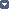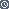## 555 Timer as an A/D converter

### By internum

I had a Basic Stamp project that needed to measure a nominal 12 volt battery, and I wanted a simple solution. This is the simplest I could come up with.555 Timer as an A/D converter circuit

The 555 timer will put out positive pulses. The pulse width is inversely proportional to the difference in voltage between the voltage at "ANALOG IN" and the voltage of the 4.7uF capacitor(let's say 2.5 volts). To calibrate this circuit, hook it up to a Basic Stamp measuring positive pulses, and give the circuit a known voltage. Let's say you get the number 2092 when you give the circuit 15 volts. Your coefficient is 2092 * (15 - 2.5) = 26150. Now you are ready to measure voltage with your Basic Stamp. Use the formula: voltage = 26150/pulse + 2.5 . You will have to modify this to work within the limits of the Basic Stamp's math. The accuracy of this circuit rivals many digital voltmeters within the range I tested it (6 volts to 18 volts), about the same as a 10 bit A/D converter. The accuracy will shift with the processor clock and the +5 supply, so it is pretty good. Conversion time is under 1/10 second. Please note it will not measure voltages below 5 volts. Also, check the accuracy of your +5 volts. If it is 5.2 volts, you will need to use 2.6 in the formula.

A sample program listing follows.

'uncomment the debug lines to get pulse value while calibrating loop: 'debug cls pulsin 0,1,w2 'I used pin 0 'debug w2 w1=26150 'This is the coefficient you will need to calibrate. w4=w1/w2 w3=w4*100 'I am going to get around the integer-only Stamp math. w4=w2*w4 w1=w1-w4*10 'remember the Stamp has left-to-right math w4=w1/w2 w3=w4*10+w3 w4=w2*w4 w1=w1-w4*10 w4=w1/w2 w3=w4+w3 w3=w3+250 '250 is really 2.5 volts debug w3,"volts * 100" 'we get a reading in hundredths of volts goto loop

The software and hardware are released into the public domain.

Warranty:
None.Q: You say I can’t measure less than 5 volts. I want to measure less than 5 volts. Can I measure less than 5 volts? A: Yes, you may measure down to 4 or 4.5 volts with dramatically reduced accuracy. Q: How can this be accurate? The frequency of the 555 will not be linear! A: It’s not measuring frequency, it’s measuring pulse width and adjusting for the reference voltage. Microcontrollers allow us to use software to compensate for simple hardware! Q: Must I use a Basic Stamp? A: No, the principle is the same no matter what microcontroller you use. The program as shown will work on a Basic Stamp 1. Q: I want to use a Basic Stamp 2. What changes should I make? A: The BS2 runs five times faster, so the simplest thing you can do is make the circuit 5 times faster. Use a 1uF capacitor instead of 4.7uF.228119 September 2009